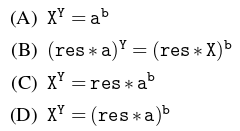# GATE | GATE-CS-2016 (Set 2) | Question 45

The following function computes XY for positive integers X and Y.

 `int` `exp``(``int` `X, ``int` `Y) ` `{ ` `    ``int` `res = 1, a = X, b = Y; ` `    ``while` `( b != 0 ) ` `    ``{ ` `        ``if` `( b%2 == 0) ` `        ``{ ` `            ``a = a*a; ` `            ``b = b/2; ` `        ``} ` `        ``else` `        ``{ ` `            ``res = res*a; ` `            ``b = b-1; ` `        ``} ` `    ``} ` `    ``return` `res; ` `} `

Which one of the following conditions is TRUE before every iteration of the loop(A) A
(B) B
(C) C
(D) D

Explanation:

We can solve this question taking any two values for X and Y.
Suppose X= 2 and Y= 5, now this code will calculate
Looking at each iteration separately
Before iteration 1 – X=2 Y= 5 a=2 , b=5, res=1
Iteration 1 – since b%2 !=0 we go to else part
Therefore after iteration 1, X=2, Y=5, a=2, b=4, res=2
Iteration 2 – since b%2=0 we go to if part
Therefore after iteration 2 , X=2, Y=5, a=4, b=2, res=2
Iteration 3 – since b%2=0 we go to if part
Therefore after iteration 3 , X=2, Y=5, a=16, b=1, res=2
Iteration 4 – since b%2!=0 we go to else part
Therefore after iteration 4 , X=2, Y=5, a=16, b=0, res=32
Now putting the values of X, Y , a, b, res in the equations given in options after each
iteration we can see only equation c is correct.

This solution is contributed by Parul sharma.

Another solution

In option C

```Before Iteration 1: X^Y=64 res ∗ (a^b)=64
Before Iteration 2: X^Y=64 res ∗ (a^b)=64
Before Iteration 3: X^Y=64 res ∗ (a^b)=64 ```
My Personal Notes arrow_drop_up
Article Tags :

Be the First to upvote.

Please write to us at contribute@geeksforgeeks.org to report any issue with the above content.